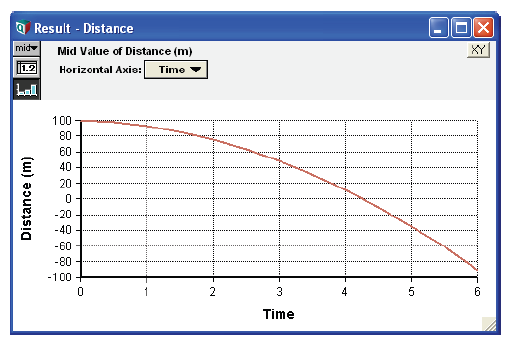# Dynamic dependencies

All variables with dynamic inputs are evaluated dynamically — that is, their results are arrays indexed by Time.

Example: A series of dynamic definitions produce equations for distance, velocity, and acceleration:

Acceleration: -9.8
Dt: 0.5
Time: Sequence(0, 6, Dt)
Velocity: Dynamic(0, Self[ Time - 1] + Acceleration*Dt)
Distance: Dynamic(100, Self[ Time - 1] + Velocity*Dt) →## Dynamic dependency arrows

If a variable is dynamically dependent on another variable, a gray arrow is drawn between the variables.

To show or hide dynamic dependency arrows:

1. Select Set Diagram Style from the Diagram menu to open the Diagram Style dialog.
2. Click in the Dynamic checkbox to show dynamic arrows (or uncheck it to hide the arrows).
3. Click OK to accept the change.

## Expressions inside dynamic loops

A dynamic loop is a sequence of variables beginning and ending at the same variable, with each consecutive variable dependent on the previous one. At least one variable in a dynamic loop is defined using the Dynamic function.

When the definition of a variable in a dynamic loop is evaluated, the definition is repeatedly evaluated in the context of Time = t (as t increments through the values of Time). The value for any identifier that appears in an expression is implicitly sliced at Time = t (unless it is explicitly offset in Time). As an example, suppose A is indexed by Time, and X is defined as:

Dynamic(0, Self[ Time - 1] + Max(A, Time))

During evaluation, A would be an atom at any given time point since it is implicitly sliced across Time. When A is not indexed by Time, Max(A, Time) simply returns A, so that the above expression is equivalent to:

Dynamic(0, Self[ Time - 1] + A)

To add the greatest value of A along Time in this expression, you must introduce an extra variable to hold the maximum value, defined simply as Max(A, Time), and ensure that the two variables do not occur in the same dynamic loop.

If you attempt to operate over the Time dimension from within a dynamic loop, Analytica issues the warning: “Encountered application of an array function over the Time index from within a dynamic loop. The semantics of this operation might be different than you expect.”